# 0708-1300/Class notes for Tuesday, October 9

## Class Notes

The notes below are by the students and for the students. Hopefully they are useful, but they come with no guarantee of any kind.

### Scanned Notes

Announcements go here

### Typed Notes - First Hour

Reminder:

1) An immersion locally looks like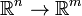$\mathbb{R}^n\rightarrow\mathbb{R}^m$ given by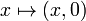$x\mapsto(x,0)$

2) A submersion locally looks like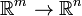$\mathbb{R}^m\rightarrow\mathbb{R}^n$ given by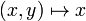$(x,y)\mapsto x$

Today's Goals

1) More about "locally things look like their differential"

2) The trick Sard's Theorem: "Evil points are rare, good points everywhere"

Definition 1

Let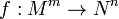$f:M^m\rightarrow N^n$ be smooth. A point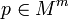$p\in M^m$ is critical if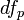$df_p$ is not onto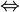$\Leftrightarrow$ rank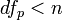$df_p . Otherwise, p is regular.

Definition 2 A point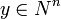$y\in N^n$ is a critical value of f if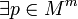$\exists p\in M^m$ such that p is critical and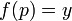$f(p) = y$. Otherwise, y is a regular value

Example 1

Consider the map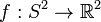$f:S^2\rightarrow\mathbb{R}^2$ given by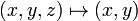$(x,y,z)\mapsto (x,y)$. I.e., the projection map. The regular points are all the points on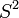$S^2$ except the equator. The regular values, however, are all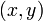$(x,y)$ such that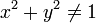$x^2+y^2 \neq 1$

Example 2

Consider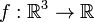$f:\mathbb{R}^3\rightarrow\mathbb{R}$ given by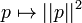$p\mapsto ||p||^2$. That is,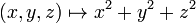$(x,y,z)\mapsto x^2+y^2+z^2$. Clearly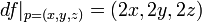$df|_{p=(x,y,z)} = (2x,2y,2z)$ and so p is regular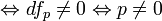$\Leftrightarrow df_p\neq 0 \Leftrightarrow p\neq 0$

So, the critical values are the image of zero, thus only zero. All other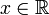$x\in\mathbb{R}$ are regular values.

Note: In both the last two examples there were points in the target space that were NOT hit by the function and thus are vacuously regular. In the previous example these are the point x<0.

Example 3

Consider a function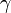$\gamma$ from a segment in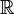$\mathbb{R}$ onto a curve in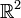$\mathbb{R}^2$ such that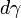$d\gamma$ is never zero. Thus, rank(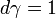$d\gamma = 1$) and so$d\gamma$ is never onto. Hence, ALL points are critical in the segment. The points on the curve are critical values, as they are images of critical points, and all points in$\mathbb{R}^2$ NOT on the curve are vacuously regular.

Theorem 1

Sard's Theorem

Almost every$y\in N^n$ is regular$\Leftrightarrow$ the set of critical values of f is of measure zero.

Note: The measure is not specified (indeed, for a topological space there is no canonical measure defined). However the statement will be true for any measure.

Theorem 2

If$f:M^m\rightarrow N^n$ is smooth and y is a regular value then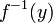$f^{-1}(y)$ is an embedded submanifold of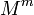$M^m$ of dimension m-n.

Re: Example 2$f^{-1}(y)$ is a sphere and hence (again!) the sphere is a manifold

Re: Example 3$f^{-1}(y)$ for regular y is empty and hence we get the trivial result that the empty set is a manifold

Proof of Theorem 2

Let$f:M^m\rightarrow N^n$ is smooth and y is a regular value. Pick a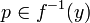$p\in f^{-1}(y)$. p is a regular point and thus$df_p$ is onto. Hence, by the submersion property (Reminder 2) we can find a "good charts" thats maps a neighborhood U of p by projection to a neighborhood V about y. Indeed, on U f looks like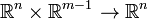$\mathbb{R}^n\times\mathbb{R}^{m-1}\rightarrow \mathbb{R}^n$ by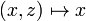$(x,z)\mapsto x$.

So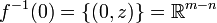$f^{-1}(0) = \{(0,z)\} = \mathbb{R}^{m-n}$. Q.E.D

Diversion

Arbitrary objects can be described in two ways:

1) With a constructive definition

2) with an implicit definition

For example, a constructive definition of lines in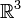$\mathbb{R}^3$ is given by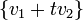$\{v_1 + tv_2\}$ but implicitly they are the solutions to the equations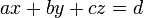$ax+by+cz = d$ and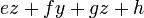$ez+fy+gz+h$.

Hence in general, a constructive definition can be given in terms of an image and an implicit definition can be given in terms of a kernal.

Homological algebra is concerned with the difference between these philosophical approaches.

Remark

For submanifolds of smooth manifolds, there is no difference between the methods of definition.

Definition 3

Loosely we have the idea that a concave and convex curve which just touch at a tangent point is a "bad" intersection as it is unstable under small perturbation where as the intersection point in an X (thought of as being in$\mathbb{R}^2$) is a "good" intersection as it IS stable under small perturbations.

Precisely,

Let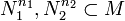$N_1^{n_1}, N_2^{n_2} \subset M$ be smooth submanifolds. Let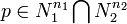$p\in N_1^{n_1} \bigcap N_2^{n_2}$

We say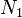$N_1$ is transverse to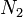$N_2$ in M at p if for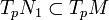$T_p N_1\subset T_p M$ and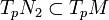$T_p N_2 \subset T_p M$ satisfies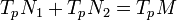$T_p N_1 + T_p N_2 = T_p M$

Example 4

Our concave intersecting with convex curve example intersecting tangentially has both of their tangent spaces at the intersection point being the same line and thus does not intersect transversally as the sum of the tangent spaces is not all of$\mathbb{R}^2$.

Our X example does however work.

### Typed Notes - Second Hour

Coming soon to a wikipedia near you.